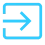Solved

# recalculate grid values (Totals)

• 4 replies
• 76 views

• Varsity I
• 28 replies

Hi good night.

`I have a problem. I need to re-calculate the values ​​of the “Accumulated amount” column every time I make a change in any column of the grid (specially the colum TotalAmount). re-calculated de values and show in the grid.`
`The business rule for the “Accumulated total amount” column is as follows:sum current row + previous row. (in the first row the previous row is zero).`
`I used this code in the row_updated event but it doesn't work as I expect. (This code is in the Row_Updated Event`
``//In this code for each interaction 1 is added so if I have a grid with 4 rows in AccumulatedAmount //it should look like: 1,2,3,4 but it doesn't work          int cont = 0;            foreach (CEEstimateAddOn soLine in this.viewCEEstimateAddOn.Select())        {          cont+=1;          soLine.AccumulatedAmount = cont;        }``
`with the code above only the “edited/active” row show the result. `
`and not in all rows as I need`
`Could you please help me.`
icon

Best answer by Naveen B 1 September 2021, 08:00

View original

### 4 replies

Userlevel 7+10

Hi @eddiedaco

`Accumulated total amount = (Current Row of Total Amount + Previous Row of Accumulated total amount)`

For this requirement, your grid should have the soring order like (LineNbr will be like 1,2,3..etc for each record). Based on this LineNbr you can fetch the proper values for “Current Row of Total Amount” and “Previous Row of Accumulated total amount” and assign it to Accumulated total amount.

HI Naveen,

I’m new to acumatica. Can you please share the code for this Accumulated total amount calculation.

Thank you..

Regards,

Ramya Krishna

Userlevel 7+10

Hi @ramya15  Can you please let me know what is your requirement and it will better that if you create a new question so that others can read provide you the solution.

Hi @Naveen B ,

I have a requirement to calculate the “Cumulative completion” based  for each task of the project based on the previous “Completion” entry.

Could you please guide me on this.